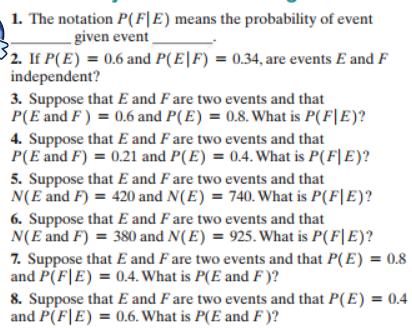# 1. The notation P(F|E) means the probability of event - given event 2. If P(E)0.6 and P(E|F) = 0.34, are events E and F independent? 3. Suppose that E and F are two events and that P(E and F) 0.6 and P(E) = 0.8. What is P(F|E)? 4. Suppose that E and F are two events and that P(E and F) 0.21 and P(E) = 0.4. What is P(F|E)? 5. Suppose that E and F are two events and that N(E and F) 420 and N(E) 740. What is P(F|E)? 6. Suppose that E and F are two events and that N(E and F) 380 and N(E) = 925. What is P(F|E)? 7. Suppose that E and F are two events and that P(E) = 0.8 and P(F E) 0.4. What is P(E and F )? 8. Suppose that E and F are two events and that P(E) = 0.4 and P(FE) 0.6. What is P(E and F)?

Question

Thank youhelp_outlineImage Transcriptionclose1. The notation P(F|E) means the probability of event - given event 2. If P(E)0.6 and P(E|F) = 0.34, are events E and F independent? 3. Suppose that E and F are two events and that P(E and F) 0.6 and P(E) = 0.8. What is P(F|E)? 4. Suppose that E and F are two events and that P(E and F) 0.21 and P(E) = 0.4. What is P(F|E)? 5. Suppose that E and F are two events and that N(E and F) 420 and N(E) 740. What is P(F|E)? 6. Suppose that E and F are two events and that N(E and F) 380 and N(E) = 925. What is P(F|E)? 7. Suppose that E and F are two events and that P(E) = 0.8 and P(F E) 0.4. What is P(E and F )? 8. Suppose that E and F are two events and that P(E) = 0.4 and P(FE) 0.6. What is P(E and F)? fullscreen

### Want to see this answer and more?

Experts are waiting 24/7 to provide step-by-step solutions in as fast as 30 minutes!*

*Response times may vary by subject and question complexity. Median response time is 34 minutes for paid subscribers and may be longer for promotional offers.
Tagged in
Math
Statistics

### Other